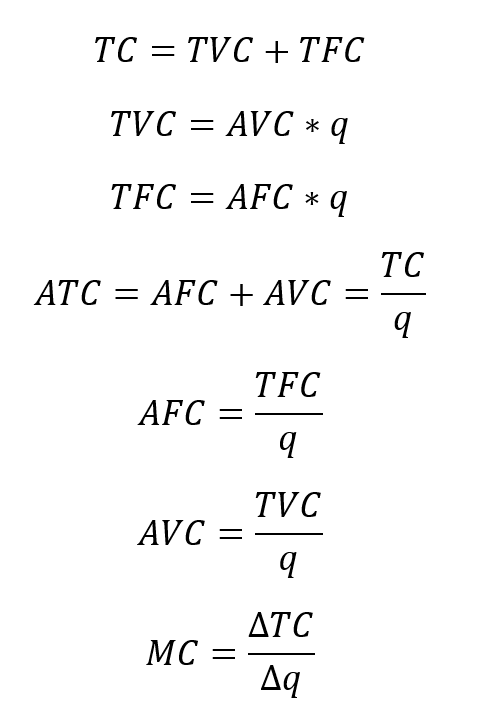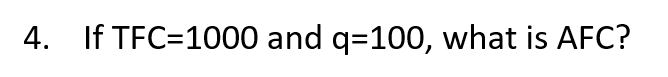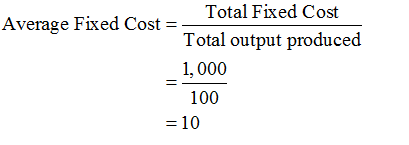# TC = TVC + TFCTVC = AVC * qTFC = AFC * qTCATC = AFC + AVCTFCAFC =TVCAVC =ATCMC =Да 4. If TFC=1000 and q=100, what is AFC?

Question
2 viewshelp_outlineImage TranscriptioncloseTC = TVC + TFC TVC = AVC * q TFC = AFC * q TC ATC = AFC + AVC TFC AFC = TVC AVC = ATC MC = Да fullscreenhelp_outlineImage Transcriptionclose4. If TFC=1000 and q=100, what is AFC? fullscreen
check_circle

Step 1

The cost of production is the summation of costs incurred in the process of producing the commodity. It includes the cost of raw materials used in the production, labor used, technology used and capital used etc. The summation of all the costs incurred during the process of production is known as the costs of production.

Step 2

There are mainly two different types of costs such as the fixed cost and the variable cost. The fixed cost is the cost that remains the same at all the levels of output. Even when the quantity of output produced is zero, the fixed cost would be the same. This is why the fixed cost is said to be free from quantity. The variable cost is the cost of production that changes as the quantity increases. When the quantity is zero, the variable cost will be also zero. The variable cost is thus related to the quantity of output in the economy.

Step 3

It is given that the total fixed cost of production is \$1,000 and the quantity produced is 100. The average fixed cost of production can be calculated by dividing the total fixed cost with the quantity produced as follows:...

### Want to see the full answer?

See Solution

#### Want to see this answer and more?

Solutions are written by subject experts who are available 24/7. Questions are typically answered within 1 hour.*

See Solution
*Response times may vary by subject and question.
Tagged in